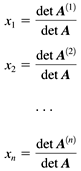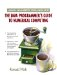# 11.6 Comparing Solution AlgorithmsJava Number Cruncher: The Java Programmer's Guide to Numerical Computing By Ronald  Mak Table of Contents Chapter  11.   Matrix Inversion, Determinants , and Condition Numbers

11.6 Comparing Solution Algorithms

In Program 11-2, we return to our original system of linear equations from the beginning of Chapter 10:whose correct solution is x 1 = 1, x 2 = -2, x 3 = 3, and x 4 = -1. The program solves this system using LU decomposition followed by forward and back substitution. Then, to compare, it solves the system using the matrix inverse and by using determinants with Cramer's rule. See Listing 11-2.

##### Listing 11-2 Comparison of algorithms for solving a system of linear equations.
` package numbercruncher.program11_2; import java.util.Random; import numbercruncher.matrix.*; /**  * PROGRAM 11-2: Compare Solution Algorithms  *  * Compare algorithms for solving a system of linear equations.  */ public class CompareSolutions {     /**      * Run the test.      * @param A the coefficient matrix      * @param b the right-hand-side vector      * @param correct the known correct solution vector      * @throws matrix.MatrixException if an error occurred      */     private void run(InvertibleMatrix A, ColumnVector b,                      ColumnVector correct)         throws MatrixException     {         System.out.println("Coefficient matrix A");         A.print(14);         System.out.print("\nb =");         b.print();         // Solve the system using LU decomposition         // with iterative improvement.         ColumnVector x = A.solve(b, true);         System.out.println("\nLU decomposition:");         System.out.print("   x =");         x.print();         System.out.println("Error vector norm = " +                            x.subtract(correct).norm());         InvertibleMatrix Ainv = A.inverse();         System.out.println("\nA inverse");         Ainv.print(14);         float detA  = A.determinant();         float condA = A.norm()*Ainv.norm();         // Solve the system by multiplying A-inverse by b.         x = Ainv.multiply(b);         System.out.println("\nMultiplication by inverse:");         System.out.print("   x =");         x.print();         System.out.println("Error vector norm = " +                            x.subtract(correct).norm());         System.out.println("\n     Determinant of A = " + detA);         System.out.println("Condition number of A = " + condA);         int              nRows  = A.rowCount();         InvertibleMatrix As[]   = new InvertibleMatrix[nRows];         float            dets[] = new float[nRows];         // Loop to create matrices A(i) for Cramer's rule.         for (int i = 0; i < nRows; ++i) {             As[i] = new InvertibleMatrix(A.copyValues2D());             As[i].setColumn(b, i);             dets[i] = As[i].determinant();             System.out.println("\nA[" + (i+1) + "], determinant = " +                                dets[i]);             As[i].print(14);         }         // Solve the system using Cramer's rule.         x = new ColumnVector(nRows);         for (int i = 0; i < nRows; ++i) x.set(i, dets[i]/detA);         System.out.println("\nCramer's rule:");         System.out.print("   x =");         x.print();         System.out.println("Error vector norm = " +                            x.subtract(correct).norm());     }     /**      * Main.      * @param args the array of arguments      */     public static void main(String args[])     {         // Matrix A.         InvertibleMatrix A = new InvertibleMatrix(new float[][] {             { 3,  1, -5,  4},             { 2, -3,  3, -2},             { 5, -3,  4,  1},             {-2,  4, -3, -5},         });         // Column vector b.         ColumnVector b =                     new ColumnVector(new float[] {-18, 19, 22, -14});         // The known correct solution.         ColumnVector correct =                     new ColumnVector(new float[] {1, -2, 3, -1});         CompareSolutions compare = new CompareSolutions();         try {             compare.run(A, b, correct);         }         catch(MatrixException ex) {             System.out.println("*** ERROR: " + ex.getMessage());             ex.printStackTrace();         }     } } `

Output:

` Coefficient matrix A Row  1:           3.0           1.0          -5.0           4.0 Row  2:           2.0          -3.0           3.0          -2.0 Row  3:           5.0          -3.0           4.0           1.0 Row  4:          -2.0           4.0          -3.0          -5.0 b =  -18.0  19.0  22.0  -14.0 LU decomposition:    x =  1.0  -2.0  3.0  -1.0 Error vector norm = 0.0 A inverse Row  1:    0.08121827   -0.02538071    0.20812182    0.11675127 Row  2:   -0.12436548   -0.49238577    0.33756346    0.16497461 Row  3:   -0.19035533   -0.28426397    0.23096447   0.007614213 Row  4:  -0.017766498   -0.21319798    0.04822335   -0.11928934 Multiplication by inverse:    x =  0.9999999  -1.9999995  2.9999998  -1.0000004 Error vector norm = 6.529362E-7      Determinant of A = 394.00003 Condition number of A = 11.255114 A, determinant = 393.99997 Row  1:         -18.0           1.0          -5.0           4.0 Row  2:          19.0          -3.0           3.0          -2.0 Row  3:          22.0          -3.0           4.0           1.0 Row  4:         -14.0           4.0          -3.0          -5.0 A, determinant = -787.99994 Row  1:           3.0         -18.0          -5.0           4.0 Row  2:           2.0          19.0           3.0          -2.0 Row  3:           5.0          22.0           4.0           1.0 Row  4:          -2.0         -14.0          -3.0          -5.0 A, determinant = 1182.0 Row  1:           3.0           1.0         -18.0           4.0 Row  2:           2.0          -3.0          19.0          -2.0 Row  3:           5.0          -3.0          22.0           1.0 Row  4:          -2.0           4.0         -14.0          -5.0 A, determinant = -394.00003 Row  1:           3.0           1.0          -5.0         -18.0 Row  2:           2.0          -3.0           3.0          19.0 Row  3:           5.0          -3.0           4.0          22.0 Row  4:          -2.0           4.0          -3.0         -14.0 Cramer's rule:    x =  0.9999998  -1.9999996  2.9999998  -1.0 Error vector norm = 4.6552717E-7 `

LU decomposition, followed by forward and back substitution, produced the exact solution. Computing x = A - 1 b introduced some errors, as did Cramer's rule.

Cramer's rule is computationally very expensive. First, we compute det A. Next, we create the matrices A (1) , A (2) , . . . , A ( n ) , where A (1) is matrix A with its first column replaced by b, A (2) is A with its second column replaced by b and so on. Then, we compute the determinant of each of the matrices A ( i ) , which means we must perform LU decomposition on each of them. Finally, the solution isThe output in Listing 11-2 shows that cond A is about 11.3, which means that A is well-conditioned, and so we shouldn't be too surprised that LU decomposition followed by forward and back substitution computes such a good solution. In general, this algorithm will do better than using the matrix inverse or Cramer's rule.TopJava Number Cruncher: The Java Programmers Guide to Numerical Computing
ISBN: 0130460419
EAN: 2147483647
Year: 2001
Pages: 141
Authors: Ronald Mak

Similar book on Amazon